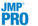Launch the Formula Depot by selecting Analyze > Predictive Modeling > Formula Depot.
Figure 11.3 Empty Formula Depot from LaunchPlatforms That Publish Prediction Formulas to the Formula Depot
 • Discriminant
 • Least Squares Regression
 • Logistic Regression
 • Partition
 • Uplift
 • K Nearest Neighbors
 • Naive Bayes
 • Neural
 • Latent Class Analysis
 • Principal Components
 • Generalized Regression
 • PLS
 • Gaussian Process

Help created on 3/19/2020